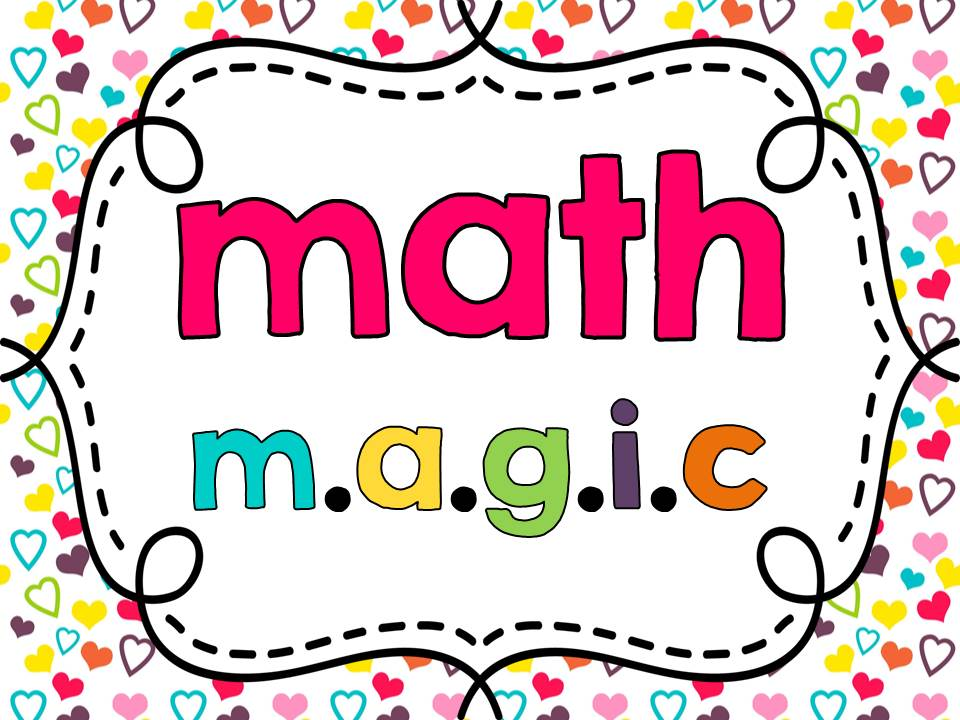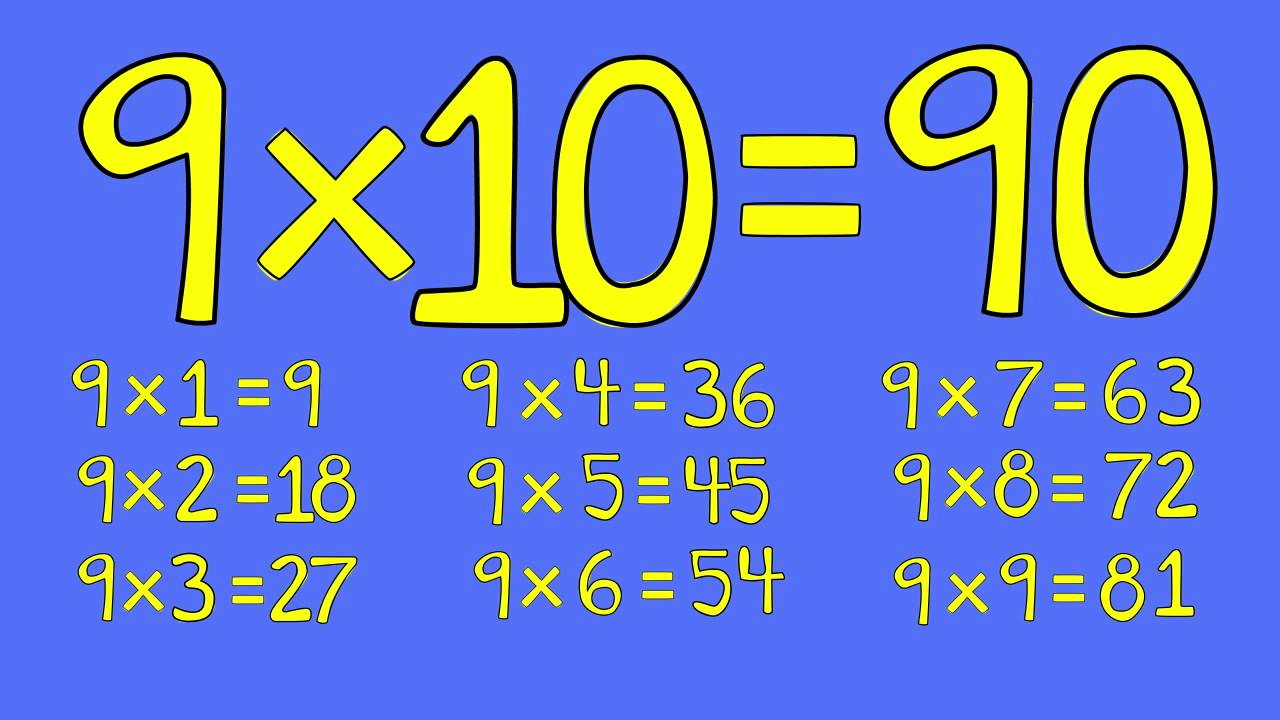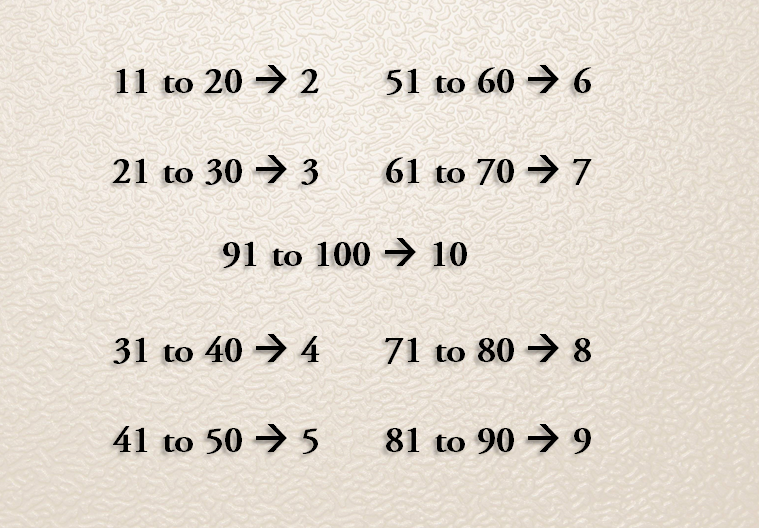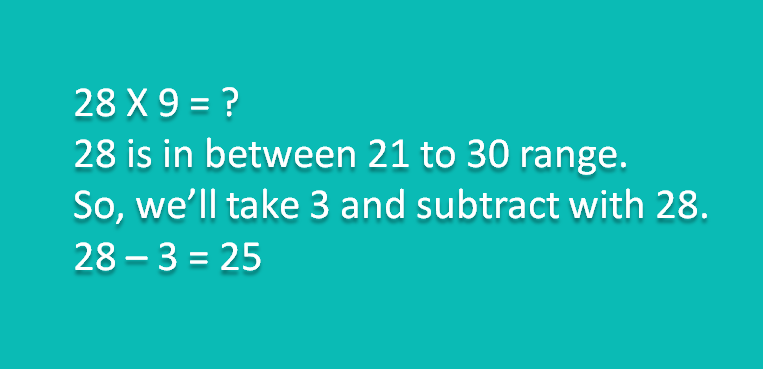## Magic Multiply by 9 Trick – Mind Calculation in 3 secHello students, I am going to start a new era of study tricks and if you’re willing to get some useful tricks then stay with us. I’ll share some useful tutorials which can help you in your competitive exams.

So in our first class i’ll teach you How to Magic multiply by 9 from 11 to 99 with mind calculation in 3 sec. Now let’s start

### Step 1 : Learn basic multiple of 9:

First you’ve to care that you should have aware the table of 9 from 1 to 9 like:Note :  We only need unit place of table 9 i.e. 9X6 = 54 then we’ll take only 4.

Are you confused? Then don’t worry let’s move on the next step. Your confusion will be gone soon.

## Step 2 : Take care of magic term

Now when you’re ready for calculation you’ve to take care of one last and important thing and it is the main part of this magic.#### What is in above image?

Actually whenever you’ve to multiple by 9 with any number from 11 to 99 then you’ll subtract that number with above corresponding range digit.

For example:Let’s take some more example so you’re aware with this important part:

• 57 X 9 = ? then 57 is in range between 51 to 60 so subtract with 6 and 57 – 6 = 51.
• 97 X 9 =? then 97 – 10 = 87
• 27 X 9 =? then 27 – 3 = 24

Note : You can remember this part by just think the last 10 multiple of that given number i.e 47 is number which we’ve to multiply and their last 10 multiple is 50 so subtract 47 with 5.

### Step 3 : Let’s multiply with magic

Now you’re ready to multiply any number with 9 in 3 sec.

28 X 9 = ?

From Step 2, we’ve to subtract 28 with 3 :

Now from Step 1, we’ve to multiply the unit place of 28 by 9 i.e. 8 X 9.

8 X 9 = 72 and we’ll take only unit place of answer i.e. 2

28 X 9 = 252

Let’s take one more example : 57 X 9 =?

57 – 6 = 51

7 X 9 = 63

57 X 9 = 513

### Step 4 : Let’s practice by below quiz#### By Tell Me How

It is a technology blog and admin has excellent experience in programming from 5+ year. You can contact us at ceo.tellmehow@gmail.com

#### 6 Incredible Ways Technology is Transforming the Service